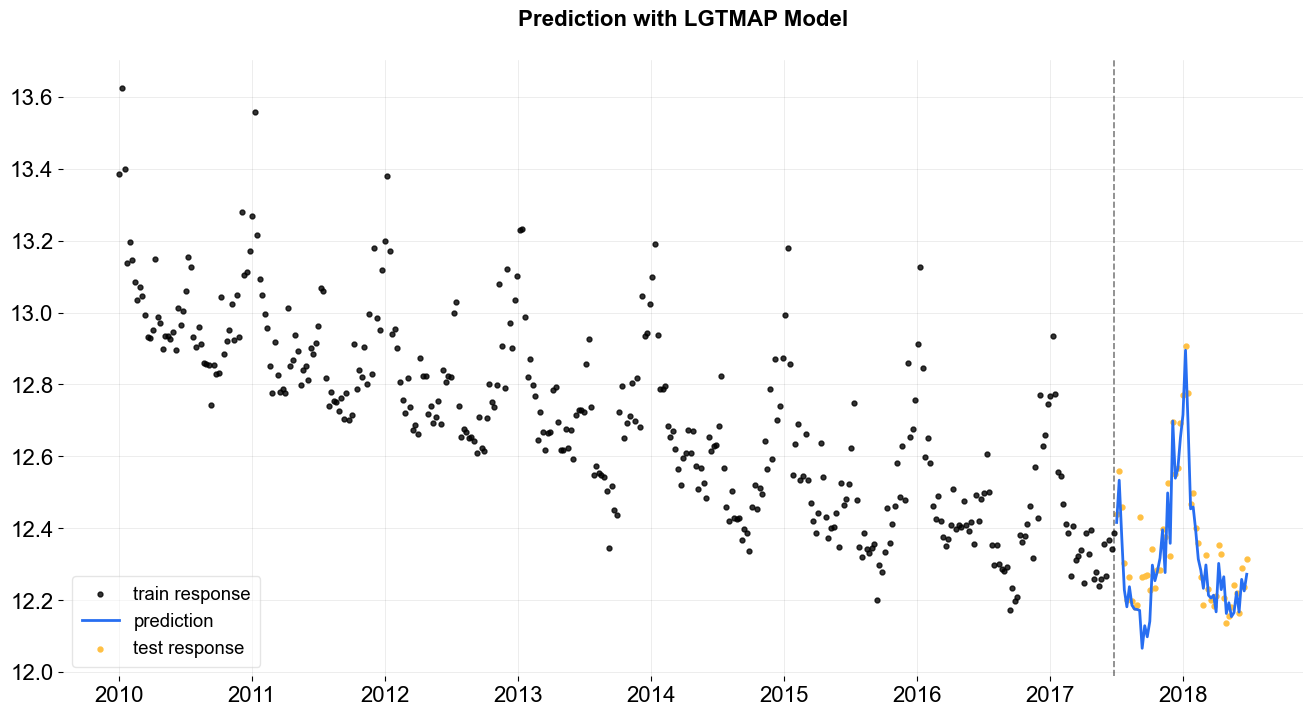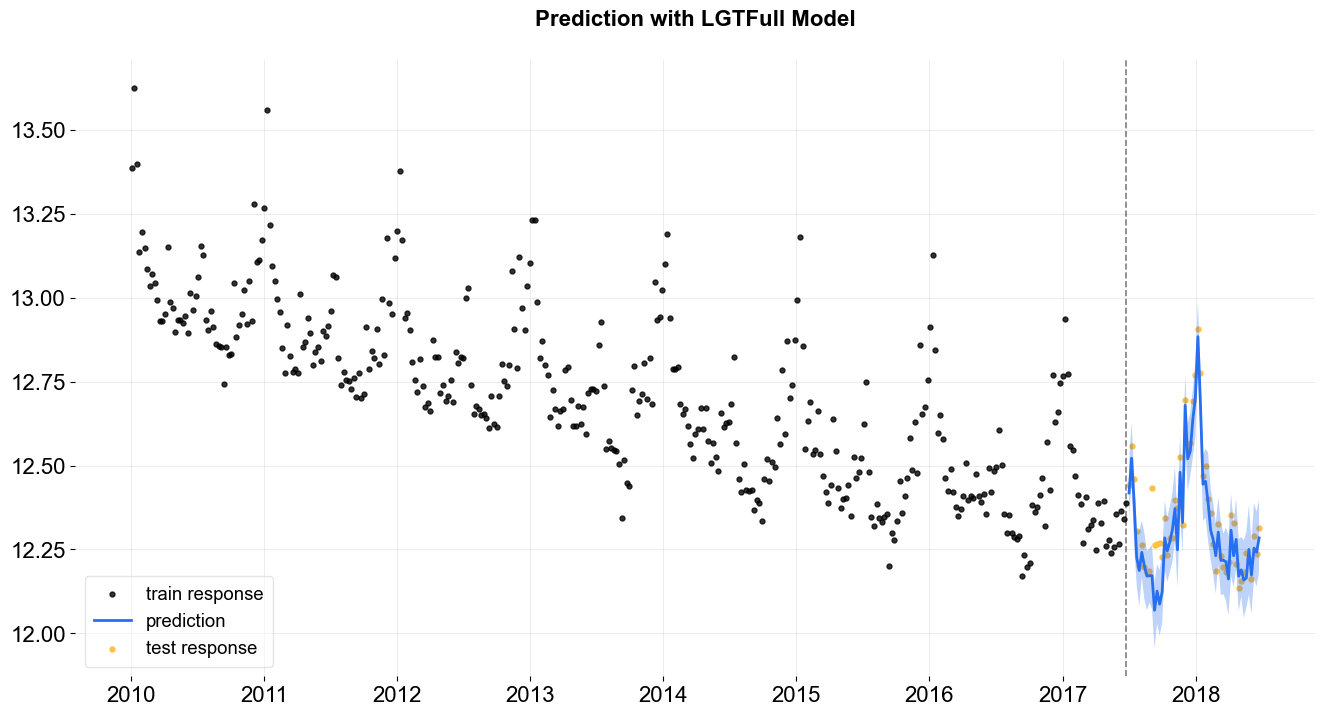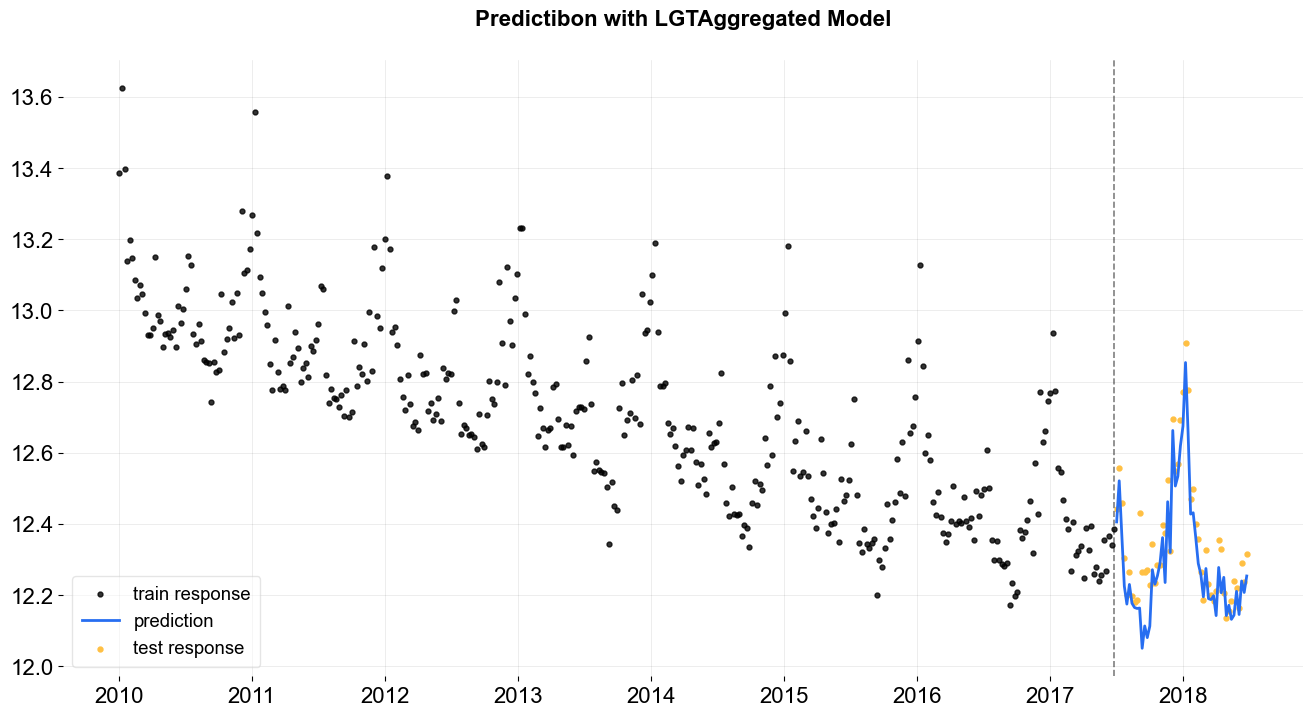# Local Global Trend (LGT)¶

In this section, we will cover:

• LGT model structure

• difference between DLT and LGT

• syntax to call LGT classes with different estimation methods

LGT stands for Local and Global Trend and is a refined model from Rlgt (Smyl et al., 2019). The main difference is that LGT is an additive form taking log-transformation response as the modeling response. This essentially converts the model into a multiplicative with some advantages (Ng and Wang et al., 2020). However, one drawback of this approach is that negative response values are not allowed due to the existence of the global trend term and because of that we start to deprecate the support of regression of this model.

:

%matplotlib inline

import pandas as pd
import numpy as np
import matplotlib.pyplot as plt

import orbit
from orbit.models import LGT
from orbit.diagnostics.plot import plot_predicted_data
from orbit.diagnostics.plot import plot_predicted_components

:

print(orbit.__version__)

1.1.4


## Model Structure¶

\begin{split}\begin{align*} y_{t} &= \mu_t + s_t + \epsilon_t \\ \mu_t &= l_{t-1} + \xi_1 b_{t-1} + \xi_2 l_{t-1}^{\lambda}\\ \epsilon_t &~\sim \mathtt{Student}(\nu, 0, \sigma)\\ \sigma &~\sim \mathtt{HalfCauchy}(0, \gamma_0) \end{align*}\end{split}

with the update process,

\begin{split}\begin{align*} l_t &= \rho_l(y_t - s_t) + (1-\rho_l)l_{t-1}\\ b_t &= \rho_b(l_t - l_{t-1}) + (1-\rho_b)b_{t-1}\\ s_{t+m} &= \rho_s(y_t - l_t ) + (1-\rho_s)s_t \end{align*}\end{split}

Unlike DLT model which has a deterministic trend, LGT introduces a hybrid trend where it consists of

• local trend takes on a fraction $$\xi_1$$ rather than a damped factor

• global trend is with a auto-regrssive term $$\xi_2$$ and a power term $$\lambda$$

We will continue to use the iclaims data with 52 weeks train-test split.

:

# load data
# define date and response column
date_col = 'week'
response_col = 'claims'
df.dtypes
test_size = 52
train_df = df[:-test_size]
test_df = df[-test_size:]


## LGT Model¶

In orbit, we provide three methods for LGT model estimation and inferences, which are * MAP * MCMC (also providing the point estimate method, mean or median), which is also the default * SVI

Orbit follows a sklearn style model API. We can create an instance of the Orbit class and then call its fit and predict methods.

In this notebook, we will only cover MAP and MCMC methods. Refer to this notebook for the pyro estimation.

### LGT - MAP¶

To use MAP, specify the estimator as stan-map.

:

lgt = LGT(
response_col=response_col,
date_col=date_col,
estimator='stan-map',
seasonality=52,
seed=8888,
)

2023-01-20 18:07:04 - orbit - INFO - Optimizing (PyStan) with algorithm: LBFGS.

:

%%time
lgt.fit(df=train_df)

CPU times: user 35.9 ms, sys: 7.82 ms, total: 43.7 ms
Wall time: 91.1 ms

:

<orbit.forecaster.map.MAPForecaster at 0x2932212b0>

:

predicted_df = lgt.predict(df=test_df)

:

_ = plot_predicted_data(training_actual_df=train_df, predicted_df=predicted_df,
date_col=date_col, actual_col=response_col,
test_actual_df=test_df, title='Prediction with LGTMAP Model')### LGT - MCMC¶

To use MCMC sampling, specify the estimator as stan-mcmc (the default).

• By dedault, full Bayesian samples will be used for the predictions: for each set of parameter posterior samples, the prediction will be conducted once and the final predictions are aggregated over all the results. To be specific, the final predictions will be the median (aka 50th percentile) along with any additional percentiles provided. One can use .get_posterior_samples() to extract the samples for all sampling parameters.

• One can also specify point_method (either mean or median) via .fit to have the point estimate: the parameter posterior samples are aggregated first (mean or median) then conduct the prediction once.

#### LGT - full¶

:

lgt = LGT(
response_col=response_col,
date_col=date_col,
seasonality=52,
seed=8888,
)

:

%%time
lgt.fit(df=train_df)

2023-01-20 18:07:04 - orbit - INFO - Sampling (PyStan) with chains: 4, cores: 8, temperature: 1.000, warmups (per chain): 225 and samples(per chain): 25.


CPU times: user 198 ms, sys: 103 ms, total: 301 ms
Wall time: 4.59 s

:

<orbit.forecaster.full_bayes.FullBayesianForecaster at 0x1768b49d0>

:

predicted_df = lgt.predict(df=test_df)

:

predicted_df.tail(5)

:

week prediction_5 prediction prediction_95
47 2018-05-27 12.118799 12.228815 12.330452
48 2018-06-03 12.075659 12.165414 12.329612
49 2018-06-10 12.128487 12.266566 12.380391
50 2018-06-17 12.130710 12.229549 12.336709
51 2018-06-24 12.179993 12.274867 12.406179
:

lgt.get_posterior_samples().keys()

:

dict_keys(['l', 'b', 'lev_sm', 'slp_sm', 'obs_sigma', 'nu', 'lgt_sum', 'gt_pow', 'lt_coef', 'gt_coef', 's', 'sea_sm', 'loglk'])

:

_ = plot_predicted_data(training_actual_df=train_df, predicted_df=predicted_df,
date_col=lgt.date_col, actual_col=lgt.response_col,
test_actual_df=test_df, title='Prediction with LGTFull Model')#### LGT - point estimate¶

:

lgt = LGT(
response_col=response_col,
date_col=date_col,
seasonality=52,
seed=8888,
)

:

%%time
lgt.fit(df=train_df, point_method='mean')

2023-01-20 18:07:09 - orbit - INFO - Sampling (PyStan) with chains: 4, cores: 8, temperature: 1.000, warmups (per chain): 225 and samples(per chain): 25.


CPU times: user 239 ms, sys: 45.5 ms, total: 285 ms
Wall time: 5.17 s

:

<orbit.forecaster.full_bayes.FullBayesianForecaster at 0x176980fa0>

:

predicted_df = lgt.predict(df=test_df)

:

predicted_df.tail(5)

:

week prediction
47 2018-05-27 12.200619
48 2018-06-03 12.134535
49 2018-06-10 12.228683
50 2018-06-17 12.196195
51 2018-06-24 12.242170
:

_ = plot_predicted_data(training_actual_df=train_df, predicted_df=predicted_df,
date_col=lgt.date_col, actual_col=lgt.response_col,
test_actual_df=test_df, title='Predictibon with LGTAggregated Model')